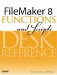# Time()

Category: Time

 Syntax: Time ( hours; minutes; seconds )

Parameters:

hoursA number representing the hours portion of the desired time value.

minutesA number representing the minutes portion of the desired time value.

secondsA number representing the seconds portion of the desired time value.

Data type returned: Time

Description:

Returns a time value built from the specified hours, minutes, and seconds parameters. The resulting value accurately calculates the effect of fractional parameters. Similarly, although the typical range for the minutes and seconds parameters is from 0 to 59, any values above or below are compensated for in the resulting time value.

The Time() function is often used in conjunction with the Hour(), Minute(), and Seconds() functions. For instance, the following formula takes the current time and returns the time of the next lowest hour:

``` Time (Hour (Get (CurrentTime));0;0))
```

Examples:

Function

Results

Time (8; 34; 15)

Returns 8:34:15.

Time (15.25; 0; 0 )

Returns 15:15:00.

Time (22; 70; 70 )

Returns 23:11:10.

Time (12; -30; 0)

Returns 11:30:00.FileMaker 8 Functions and Scripts Desk Reference
ISBN: 0789735113
EAN: 2147483647
Year: 2004
Pages: 352